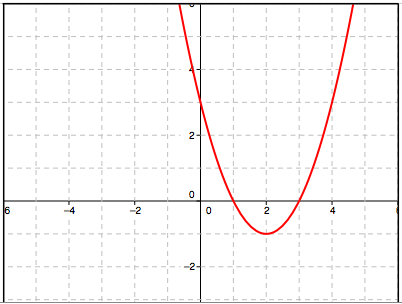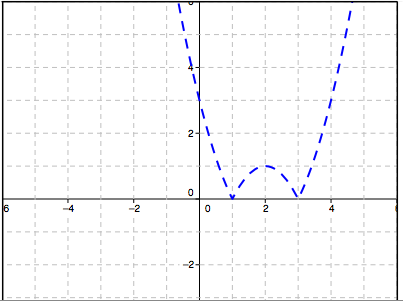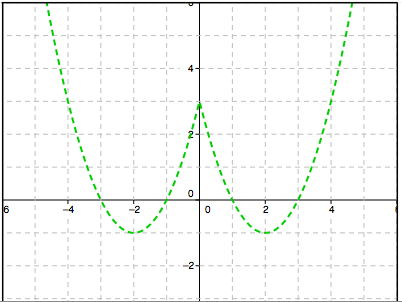### Home > A2C > Chapter 12 > Lesson 12.5.2 > Problem12-214

12-214.
1. Sketch the graph of g(x) = (x − 2)2 −1. Homework Help ✎

1. On the same set of axes (in a different color) sketch the graph of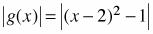.

2. On a new set of axes sketch the graph of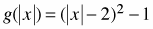.

3. Describe how each of the graphs in parts (a) and (b) are related to the original graph of g(x).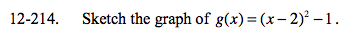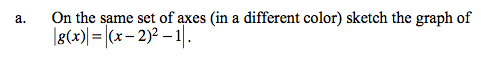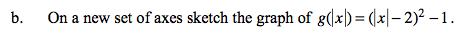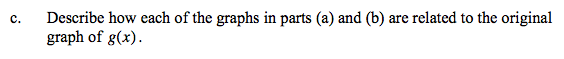Where are the graphs the same? Where are they different?